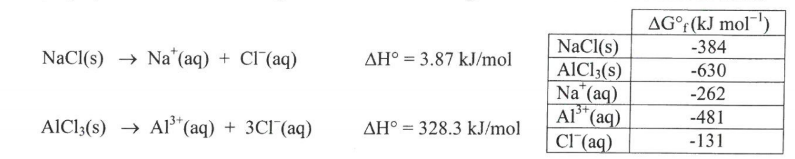# Problem: Consider the following reactions for dissolving two different chloride salts in water:  a. Calculate ΔG° for both of these reactions using the thermodynamic parameters below (assume 298K).       b. Using the results of the above calculations, what is the Ksp of these salts?     c. Which salt is more soluble? AlCl3(s) or NaCl(s)   d. Assuming each solution reaches saturation, which salt should I add to increase the boiling point of water more? AlCl3(s) or NaCl(s)

###### Problem Details

Consider the following reactions for dissolving two different chloride salts in water:

a. Calculate ΔG° for both of these reactions using the thermodynamic parameters below (assume 298K).

b. Using the results of the above calculations, what is the Ksp of these salts?

c. Which salt is more soluble?
AlCl3(s) or NaCl(s)

d. Assuming each solution reaches saturation, which salt should I add to increase the boiling point of water more?
AlCl3(s) or NaCl(s)What scientific concept do you need to know in order to solve this problem?

Our tutors have indicated that to solve this problem you will need to apply the Gibbs Free Energy concept. You can view video lessons to learn Gibbs Free Energy. Or if you need more Gibbs Free Energy practice, you can also practice Gibbs Free Energy practice problems.

What is the difficulty of this problem?

Our tutors rated the difficulty ofConsider the following reactions for dissolving two differen...as high difficulty.

How long does this problem take to solve?

Our expert Chemistry tutor, Dasha took 14 minutes and 59 seconds to solve this problem. You can follow their steps in the video explanation above.

What professor is this problem relevant for?

Based on our data, we think this problem is relevant for Professor Gokal's class at UGA.